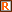You currently viewing the 2018 - 2019 Academic Catalog/Student Handbook. The current catalog is located here.

## Polynomial/Quadratic Appl NEW VERSION 2018FA

Course Course Title Class Lab/ Shop Clinical/ Co-op Credit
DMA 060 Polynomial/Quadratic Appl NEW VERSION 2018FA.75 .50 0 1
Prerequisites: Take One Set: Set 1: (DMA 010, DMA 020, DMA 030, DMA 040, and DMA 050) or Set 2: (DMA 010, DMA 020, DMA 030, and DMA 045) or Set 3: (DMA 025, DMA 040, and DMA 050) or Set 4: (DMA 025 and DMA 045)
Corequisites: None
Effective Term: Fall 2017
 This course provides a study of problems involving algebraic representations of quadratic equations. Topics include basic polynomial operations, factoring polynomials, and solving polynomial equations by means of factoring. Upon completion, students should be able to find algebraic solutions to contextual problems with quadratic applications.
 ·Competencies·. Represent real-world applications as quadratic equations.·. Apply exponent rules.·. Solve application problems involving polynomial operations.·. Apply the principles of factoring when solving problems.·. Analyze the graph of a quadratic function.·Student Learning Outcomes1. Demonstrate the use of a problem solving strategy to include multiple representations of the situation, organization of the information, and algebraic representation of quadratic equations.2. Add and subtract polynomials.3. Apply exponent rules.4. Multiply polynomials.5. Divide a polynomial by a monomial.6. Factor trinomials using multiple methods.7. Factor the difference of two squares.8. Solve quadratic applications using the zero product property and critique the reasonableness of solutions found.9. Given the graph of a parabola, identify the vertex and x-intercepts.

2015FA - New Course Version (S24128)

2018FA – New Course Version (S24985)

Legend

• : Comprehensive Articulation Agreement - This course is recommended for students transferring to a four-year university.
• : Local Requirement
• : Instructional Service Agreement
• : Universal General Education Transfer Component (UGETC)
• : Global Distinction
• : Requisite(s)